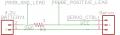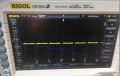# Current Measurement using oscilloscope

#### abc14

Joined Oct 15, 2017
105
Couple of days ago I wanted to measure how much current a servo motor is drawing during its operation.

I connected a 22 ohm(that is the lowest resistor i could find) resistor in series with a oscilloscope probe. My supply voltage is 4.2V, with 22 ohm resistor voltage dropped to 3.36 when servo was being actuated.

I measured that 3.36 by place the prob on the positive supply of the servo in my circuit.

So I concluded the servo current draw using ohm's law

3.36/22 = 152 mA roughly.

Just want somebody to verify, if my method of evaluating the current is valid and my calculations are making sense.

#### Danko

Joined Nov 22, 2017
849
1. You should use resistor in series with your servo.
2. Oscilloscope should be connected across resistor.
3. Then calculate current, as you did.

•-live wire-

#### dl324

Joined Mar 30, 2015
9,547
If scope ground is connected to earth ground, you must make voltage measurements with respect to circuit ground. Put the sense resistor on the low side.

You're not going to get a good measurement with the sense resistor dropping 20% of the voltage.

•Lundwall_Paul

#### abc14

Joined Oct 15, 2017
105

#### MrChips

Joined Oct 2, 2009
19,907
How many 22Ω resistors do you have?
Connect ten of them in parallel to give you 2.2Ω
Is your servo motor and drive circuitry floating? Then it doesn't matter which side of the motor the resistor is connected.

Otherwise, put the resistor in series between the motor and the GND.
Connect the oscilloscope probes in parallel across the resistor, ground clip on the probe to GND, probe at the motor-resistor connection.

#### Danko

Joined Nov 22, 2017
849
We can see current pulses 4ms with amplitude (293mV + 19.5mV)/22=14,2mA and frequency 50Hz.
Are your probe setting in oscilloscope and switch on probe the same?

Last edited:
•abc14

#### abc14

Joined Oct 15, 2017
105
We can see current pulses 4ms with amplitude (293mV + 19.5mV)/22=14,2mA and frequency 50Hz.
Are your probe setting in oscilloscope and switch on probe the same?
Yes my setting on the prob are same, only thing changed is I did manage to borrow a 1 ohm resistor. So calculations would be (293mV +19.5mV)/1.10 = 286 mA ?[/QUOTE]

Last edited by a moderator:

#### abc14

Joined Oct 15, 2017
105
How many 22Ω resistors do you have?
Connect ten of them in parallel to give you 2.2Ω
Is your servo motor and drive circuitry floating? Then it doesn't matter which side of the motor the resistor is connected.

Otherwise, put the resistor in series between the motor and the GND.
Connect the oscilloscope probes in parallel across the resistor, ground clip on the probe to GND, probe at the motor-resistor connection.
I have replaced 22 ohm resistor with a 1 ohm resistor. My circuits is belowJoined Mar 10, 2018
3,771
•BR-549

Joined Mar 10, 2018
3,771
The trace you shows looks fairly normal (for a current scaled case).

Some ref material on servos, attached.

Regards, Dana.

#### Attachments

• 160.7 KB Views: 6

#### ccdmaker

Joined Jan 1, 2017
7
This is absolutely the best way. It avoids the problem of creating a ground path through the probe - For instance, if your circuit is not isolated from AC supply ground, like it would be if it is some way connected to say, the USB port in your desktop PC , bad things can happen!

#### Danko

Joined Nov 22, 2017
849
Yes my setting on the prob are same, only thing changed is I did manage to borrow a 1 ohm resistor. So calculations would be (293mV +19.5mV)/1.10 = 286 mA ?
Right, you got it.Last edited:

#### Lundwall_Paul

Joined Oct 18, 2011
226

#### abc14

Joined Oct 15, 2017
105
Right, you got it.Thanks, although it would be nice to get some clarifications on instantaneous voltage readings so I understand (293mA and 19.20mA ) are peak to peak voltages. Are they sufficient to give good indication of current usage ? or I have to get readings over time and take the average ?

Secondly I was expecting a surge of current when the servo actuate ?

The whole point of this test is to use determine weather we could use a 250mA rated D battery for this application or not. One option would be to add a 1000 uF capacitor in parallel to the battery to compensate for the surge ?

Joined Mar 10, 2018
3,771
I think your cap would have to be bigger based on max angular change,
current draw, etc..

To calc cap (first approximation) -

Q = C x V
I = C x dV/dT
C = {I x dT ] / dV

So determine max dV you want, for the specified current. To determine
current use scope math to integrate over a cycle of waveform, and series
low value R to develop current waveform/value.

Regards, Dana.

•abc14

#### abc14

Joined Oct 15, 2017
105
I think your cap would have to be bigger based on max angular change,
current draw, etc..

To calc cap (first approximation) -

Q = C x V
I = C x dV/dT
C = {I x dT ] / dV

So determine max dV you want, for the specified current. To determine
current use scope math to integrate over a cycle of waveform, and series
low value R to develop current waveform/value.

Regards, Dana.
with regards to determining the max dv, shouldn't it be (293mV +19.50 mA) ? and Current .284mA?

#### abc14

Joined Oct 15, 2017
105
I think your cap would have to be bigger based on max angular change,
current draw, etc..

To calc cap (first approximation) -

Q = C x V
I = C x dV/dT
C = {I x dT ] / dV

So determine max dV you want, for the specified current. To determine
current use scope math to integrate over a cycle of waveform, and series
low value R to develop current waveform/value.

Regards, Dana.

These are my results using math to integrate over a cycle#### -live wire-

Joined Dec 22, 2017
901
I would use a proper shunt (.05-.35 ohms) and an op-amp to get decent readings. You can even make your own shunt using a lot of wire. Just don't wind it so that you also make an inductor. You can measure it's resistance by measuring the voltage drop at a known current (like from constant current source). If you use a higher resistance shunt than it will affect the current draw.

Joined Mar 10, 2018
3,771
The avg current is the fractional duty cycle x peak current, for purposes
of equation.

Regards, Dana.

#### MrChips

Joined Oct 2, 2009
19,907
Note that average current and rms current are not the same.
Average current is the integral (sum) of current over time, divided by the time period.

rms current is the integral of the square of the current divide by the time period, followed by the square root.